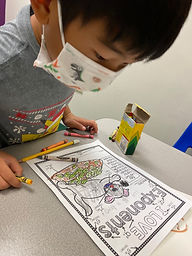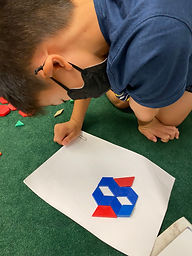## Ms. Brianne

### Target 1​

###### Lesson Type:

New

Number Operation

:

Computation

Evaluate arithmetic expressions that include an exponent.

###### 1:

Understand that an exponent indicates the number of times that a base is multiplied by itself.

###### 2:

Write a repeating multiplication equation that is equivalent to a given exponent.

###### 3:

Write an exponent that is equivalent to a given repeating multiplication equation.

6th

###### Vocabulary:

Exponent, Base, Times, Multiply

Activities:

• Students were introduced to working with exponents by creating repeating multiplication equations to match a given exponent.
• Students wrote the exponent to match a given repeating multiplication equation.
• Students solved for different exponents and used a key to color in the picture.### Home Exploration

###### Guiding Questions:## Absent Students:

Lila

### Target 2

:

###### 1:

Identify the center point of a shape.

###### 2:

Rotate a given figure at 90-degree intervals around its center point.

###### 3:

Understand that a shape has rotational symmetry if it can be turned less than 360 degrees and look exactly like the original.

4th

###### Vocabulary:

Center, Rotational Symmetry, Rotate

Activities:

• Students discussed and sorted 2D shapes by whether they had rotational symmetry or not.
• Students created their own designs that had rotational symmetry using pattern blocks.### Home Exploration

###### Guiding Questions:### Target 3

:

###### Vocabulary:

Activities:### Home Exploration